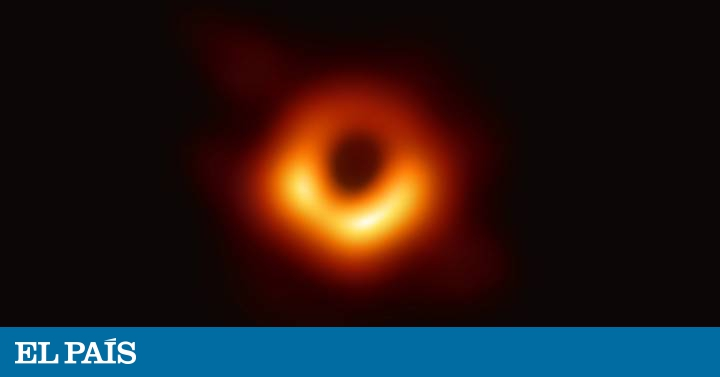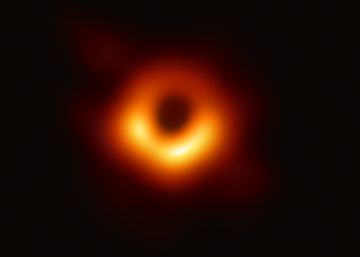Tue. Oct 22nd, 2019

Possibly the most important conjecture of general relativity | ScienceEinstein's general relativity is, today, the best and most accurate theory of existing gravity. Its publication in 1915 was a radically different way of understanding the universe. Since then, numerous scientists have launched themselves to study and find solutions for the equations of the German physicist. Each solution describes a specific space-time, and some of them sometimes contain singular points, better known as singularities, where spacetime "breaks" (the curvature becomes infinite). They are places where matter is in such extremely dense conditions that the laws of current physics are not valid, which makes a new theory capable of explaining gravity on such a small scale necessary. Physicists and mathematicians continue to try to decipher the nature of these peculiar points of the universe, which constitutes one of the greatest challenges of science.

In the real world, singularities arise in some processes of gravitational collapse, when a massive object (such as a star of sufficiently large mass) ends its fuel and begins to contract due to its own weight, concentrating more and more mass in less volume. Einstein's equations predict that, if the body has enough mass, the collapse will continue until the mass of the star is concentrated at a single point. That point is a singularity.

But where are these points in our universe? In 1969 the British scientist Roger Penrose formulated the so-called weak version of the conjecture of cosmic censorship, which holds that the singularities must be isolated from the rest of the universe inside black holes. In this way, the regions where the equations stop making sense are hidden from the view of any observer outside of them. This implies that every star that collapses and forms a singularity must end up being a black hole.

Penrose tried to refute the validity of cosmic censorship by studying the energy and the total area of ​​black holes

It was Penrose himself who in 1973 tried to refute the validity of cosmic censorship by studying the energy and the total area of ​​black holes. Specific, established an inequality relationship between these two magnitudes, which is valid as long as the cosmic censorship is true. It is a litmus test for cosmic censorship, since the existence of spacetime where such a relationship would not be fulfilled would invalidate it. So far this has happened important tests (included that of its own author) and it has not been possible to refute its validity, which has probably made it the biggest challenge that general relativity faces today.

Apart from being an interesting mathematical problem, this conjecture could have important physical consequences in the real universe. The theory of relativity is deterministic, that is, the mathematical model that describes the universe in an "instant of time" gives rise to a single future evolution of it. In other words, the information contained in a "photograph of the universe" would predetermine all the coming events.

In a universe where cosmic censorship would be violated there would be the so-called "naked" singularities, which would not be found inside black holes. These uncovered singularities could leave matter and radiation and reach us

In a universe where cosmic censorship would be violated there would be the so-called "naked" singularities, which would not be found inside black holes. From these uncovered singularities, matter and radiation could come out and reach us. Thus, for example, the trajectory of an astral object could suddenly be modified by a gravitational pulse coming out of a naked singularity, removing it from the orbit established by general relativity. With this, the relations of cause and effect of nature could be seriously altered, and relativistic determinism would crumble. The immediate consequences of our actions could be very difficult to foresee, and in that case we would be mired in tremendous uncertainty. To what extent would it make sense to talk about freedom and responsibility in our actions if our lives were subjected to extreme randomness?

Although there are mathematical models of the universe where naked singularities arise, it is not known whether in nature the conditions necessary for their formation can take place, and therefore their real existence in the universe is today a question.

"The most beautiful experience we have at our disposal is the mystery", affirmed Albert Einstein. If Penrose were right with his conjecture, all the unpredictability generated by the singularities would be locked inside the black holes, completely inaccessible, and save relativistic determinism. In this case, the only way to approach the mysteries of the singularities would be the accounts and the equations with which the human being tries to understand the cosmos.

Alberto Soria he is a postdoctoral researcher in the Institute of Mathematical Sciences (ICMAT) and is a professor of Catholic University of Ávila.

Agate Timón García- Longoria He is responsible for Communication and Disclosure of ICMAT.

Coffee and theoremsIt is a section dedicated to mathematics and the environment in which they are created, coordinated by the Institute of Mathematical Sciences (ICMAT), in which researchers and members of the center describe the latest advances in this discipline, share meeting points between mathematics and other social and cultural expressions and remember those who marked their development and knew how to transform coffee into theorems. The name evokes the definition of the Hungarian mathematician Alfred Rényi: "A mathematician is a machine that transforms coffee into theorems."

Editing and coordination: Agate Timon (ICMAT).

You can follow Matter in Facebook, Twitter, Instagram or subscribe here to our newsletter

. (tagsToTranslate) conjecture (t) important (t) relativity (t) general (t) censorship (t) cosmic (t) cosmic (t) weak (t) hold (t) star (t) sufficient (t) mass ( t) must (t) form (t) turn off (t) collapse (t) hole (t) black